# NCERT Solutions: Magnetic Effect on Electric Current Notes | Study Science Class 10 - Class 10

## Class 10: NCERT Solutions: Magnetic Effect on Electric Current Notes | Study Science Class 10 - Class 10

The document NCERT Solutions: Magnetic Effect on Electric Current Notes | Study Science Class 10 - Class 10 is a part of the Class 10 Course Science Class 10.
All you need of Class 10 at this link: Class 10

In-Text Questions

Page No: 224

Q 1. Why does a compass needle get deflected when brought near a bar magnet?
The needle of a compass is a small magnet. That’s why when a compass needle is brought near a bar magnet, its magnetic field lines interact with that of the bar magnet. Hence, a compass needle gets deflected.

Page No: 228

Q 1. Draw magnetic field lines around a bar magnet.
Magnetic field lines of a bar magnet emerge from the north pole and terminate at the south pole. Inside the magnet, the field lines emerge from the south pole and terminate at the north pole, as shown in the given figure.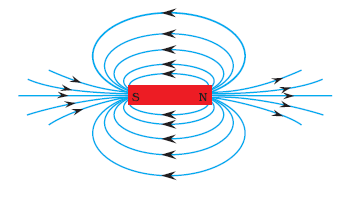Q 2. List the properties of magnetic lines of force.
The properties of magnetic lines of force are as follows:

• Magnetic field lines emerge from the north pole.
• They merge at the south pole.
• The direction of field lines inside the magnet is from the south pole to the north pole.
• Magnetic lines do not intersect with each other.

Q 3. Why don't two magnetic lines of force intersect each other?
The two magnetic field lines do not intersect each other because if they do it means at the point of intersecting the compass needle is showing two different directions which are not possible.

Page No: 229

Q 1. Consider a circular loop of wire lying in the plane of the table. Let the current pass through the loop clockwise. Apply the right-hand rule to find out the direction of the magnetic field inside and outside the loop.
Inside the loop = Pierce inside the table
Outside the loop = Appear to emerge out from the table
For downward direction of current flowing in the circular loop, the direction of magnetic field lines will be as if they are emerging from the table outside the loop and merging in the table inside the loop. Similarly, for upward direction of current flowing in the circular loop, the direction of magnetic field lines will be as if they are emerging from the table outside the loop and merging in the table inside the loop, as shown in the given figure.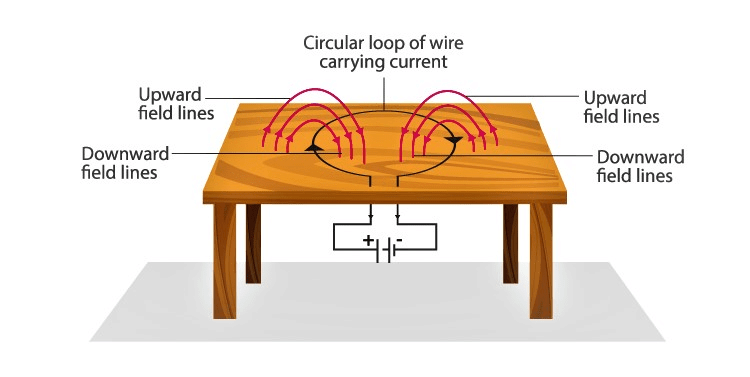Q 2. The magnetic field in a given region is uniform. Draw a diagram to represent it.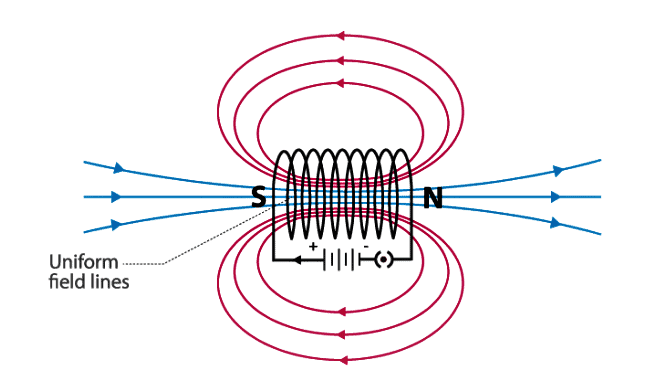The magnetic field lines inside a current-carrying long straight solenoid are uniform.

Page No: 230

Q 3. Choose the correct option.
The magnetic field inside a long straight solenoid-carrying current
(a) is zero
(b) decreases as we move towards its end
(c) increases as we move towards its end
(d) is the same at all points

Answer (d) is the same at all points

The magnetic field inside a long straight current-carrying solenoid is uniform therefore it is the same at all points.

Page No: 231

Q 1. Which of the following property of a proton can change while it moves freely in a magnetic field? (There may be more than one correct answer.)
(a) mass
(b) speed
(c) velocity
(d) momentum

Answer (c) velocity and (d) momentum

When a proton enters the region of magnetic field, it experiences magnetic force. Due to which the path of the proton becomes circular. As a result, the velocity and the momentum change.

Page No: 232

Q 2. In Activity 13.7 (page: 230), how do we think the displacement of rod AB will be affected if (i) current in rod AB is increased: (ii) a stronger horse-shoe magnet is used: and (iii) length of the rod AB is increased?
The displacement of rod AB is increased due to magnetic force exerted on it, if :
(i) If the current in the rod is increased then rod will be deflected with greater force.
(ii) If a stronger horse-shoe magnet is used then also rod will be deflected with greater force due to the increase in magnetic field.
(iii) If the length of the rod AB is increased.

Q 3. A positively-charged particle (alpha-particle) projected towards west is deflected towards north by a magnetic field. The direction of magnetic field is
(a) towards south
(b) towards east
(c) downward
(d) upward

The direction of the magnetic field can be determined using Fleming’s Left-hand rule. According to the rule, if we arrange our thumb, forefinger and the middle finger of the left-hand right perpendicular to each other, then the thumb points towards the direction of the magnetic force, the middle finger the direction of current and the forefinger the direction of the magnetic field. Since the direction of positively charged particle is towards west, the direction of the current will also be towards the west. The direction of the magnetic force is towards the north, hence the direction of the magnetic field will be upward according to Fleming’s Left-hand rule.

Page No: 233

Q 1. State Fleming's left-hand rule.
Fleming's left-hand rule states that if we arrange the thumb, the centre finger, and the forefinger of the left hand at right angles to each other, then the thumb points towards the direction of the magnetic force, the centre finger gives the direction of current, and the forefinger points in the direction of magnetic field.

Q 2. What is the principle of an electric motor?
The principle of an electric motor is based on the magnetic effect of electric current. A current-carrying loop experiences a force and rotates when placed in a magnetic field. The direction of rotation of the loop is according to Fleming’s left-hand rule.

Q 3. What is the role of the split ring in an electric motor?
The split ring in the electric motor also known as a commutator reverses the direction of current flowing through the coil after every half rotation of the coil. Due to this, the coil continues to rotate in the same direction.

Page No: 236

Q 1. Explain different ways to induce current in a coil.
Different ways to induce current in a coil are as given below :
(1) If a magnetic field is changed around a coil, an induced current is set up in the coil.
(2) If a coil is moved in a magnetic field, an induced current is set up in the coil.
(3) If a coil is rotated in a uniform magnetic field, it produces an induced current in the coil.
(4) If we take two coils and insert them over a non-conducting cylindrical roll then on changing current flowing in one coil, an induced current is obtained in the other coil.

Page No: 237

Q 1. State the principle of an electric generator.
Electric generator works on the principle of electromagnetic induction. Electricity is generated by rotating a coil inside magnetic field.

Q 2. Name some sources of direct current.
Some sources of direct current are cell, DC generator, etc.

Q 3. Which sources produce alternating current?
AC generators, power plants, etc., produce alternating current.

Q 4. Choose the correct option.
A rectangular coil of copper wires is rotated in a magnetic field. The direction of the induced current changes once in each
(a) two revolutions
(b) one revolution
(c) half revolution
(d) one-fourth revolution

When a rectangular coil is rotated in magnetic field, the direction of the induced current changes once in half revolution. As a result, the direction of the current in the coil remains the same.

Page No: 238

Q 1. Name two safety measures commonly used in electric circuits and appliances.
Two safety measures commonly used in electric circuits and appliances are

• Electric Fuse: An electric fuse is connected in series it protects the circuit from overloading and prevents it from short-circuiting.
• Proper earthing of all electric circuit in which any leakage of current in an electric appliance is transferred to the ground and people using the appliance do not get the shock.

Q 2. An electric oven of 2 kW is operated in a domestic electric circuit (220 V) that has a current rating of 5 A. What result do you expect? Explain.
Current drawn by the electric oven can be obtained by the expression,
P = VI
Where,
Current = I
Power of the oven, P = 2 kW = 2000 W
Voltage supplied, V = 220 V
I = 2000/220 V = 9.09 A
Hence, the current drawn by the electric oven is 9.09 A, which exceeds the safe limit of the circuit. The fuse element of the electric fuse will melt and break the circuit.

Q 3. What precaution should be taken to avoid the overloading of domestic electric circuits?
The precautions that should be taken to avoid the overloading of domestic circuits are as follows:

• Too many appliances should not be connected to a single socket.
• Too many appliances should not be used at the same time.
• Faulty appliances should not be connected in the circuit.
• Fuse should be connected in the circuit.

Exercise

Page No: 240

1.  Which of the following correctly describes the magnetic field near a long straight wire?
(a) The field consists of straight lines perpendicular to the wire
(b) The field consists of straight lines parallel to the wire
(c) The field consists of radial lines originating from the wire
(d) The field consists of concentric circles centred on the wire

Answer (d) The field consists of concentric circles centred on the wire

The magnetic field near a long straight wire are concentric circles. Their centers lie on the wire.

2. The phenomenon of electromagnetic induction is
(a) the process of charging a body
(b) the process of generating magnetic field due to a current passing through a coil
(c) producing induced current in a coil due to relative motion between a magnet and the coil
(d) the process of rotating a coil of an electric motor

Answer (c) producing induced current in a coil due to relative motion between a magnet and the coil

The phenomenon of inducing current in a coil due to the relative motion between the coil and the magnet is known as electromagnetic induction.

3. The device used for producing electric current is called a
(a) generator
(b) galvanometer
(c) ammeter
(d) motor

The device used for producing electric current is known as generator. Generator converts mechanical energy to electric energy.

4. The essential difference between an AC generator and a DC generator is that
(a) AC generator has an electromagnet while a DC generator has permanent magnet.
(b) DC generator will generate a higher voltage.
(c) AC generator will generate a higher voltage.
(d) AC generator has slip rings while the DC generator has a commutator.

Answer (d) AC generator has slip rings while the DC generator has a commutator.

AC generators have two rings known as the slip rings while DC generators have two half rings known as the commutator. This is main difference between AC generator and DC generator.

5. At the time of short circuit, the current in the circuit
(a) reduces substantially
(b) does not change
(c) increases heavily
(d) vary continuously

When two naked wires in the circuit come in contact with each other, the amount of current flowing in the circuit increase abruptly resulting in short circuit.

6. State whether the following statements are true or false.
(a) An electric motor converts mechanical energy into electrical energy.

An electric motor converts electrical energy into mechanical energy.

(b) An electric generator works on the principle of electromagnetic induction.

An electric generator is a device that generates electricity by rotating a coil in a magnetic field.

(c) The field at the centre of a long circular coil carrying current will be parallel straight lines.

A long circular coil is a solenoid. The magnetic field lines inside a solenoid are parallel straight lines.

(d) A wire with a green insulation is usually the live wire of an electric supply.
Live wire has red insulation cover, whereas earth wire has green insulation colour in the domestic circuits.

Q 7. List three sources of magnetic fields.
Three sources of magnetic fields are as follows:

• Current-carrying conductors
• Permanent magnets
• Electromagnets

Page No: 241

Q 8. How does a solenoid behave like a magnet? Can you determine the north and south poles of a current-carrying solenoid with the help of a bar magnet? Explain.
A solenoid is a long coil of circular loops of insulated copper wire. Magnetic field lines are produced around the solenoid when a current is allowed to flow through it. The magnetic field produced by it is similar to the magnetic field of a bar magnet. The field lines produced in a current-carrying solenoid is shown in the following figure.
Magnetic Field in current-carrying solenoidIn the above figure, when the north pole of a bar magnet is brought near the end connected to the negative terminal of the battery, the solenoid repels the bar magnet. Since like poles repel each other, the end connected to the negative terminal of the battery behaves as the north pole of the solenoid and the other end behaves as a south pole. Hence, one end of the solenoid behaves as a north pole and the other end behaves as a south pole.

Q 9. When is the force experienced by a current-carrying conductor placed in a magnetic field largest?
The force experienced by a current-carrying conductor is the maximum when the direction of current is perpendicular to the direction of the magnetic field.

Q 10. Imagine that you are sitting in a chamber with your back to one wall. An electron beam, moving horizontally from back wall towards the front wall, is deflected by a strong magnetic field to your right side. What is the direction of magnetic field?
The direction of the magnetic field is vertically downwards. The direction of current is from the front wall to the back wall because negatively charged electrons are moving from back wall to the front wall. The direction of magnetic force is rightward. Hence, using Fleming's left-hand rule, it can be concluded that the direction of magnetic field inside the chamber is downward.

Q 11. Draw a labelled diagram of an electric motor. Explain its principle and working. What is the function of a split ring in an electric motor?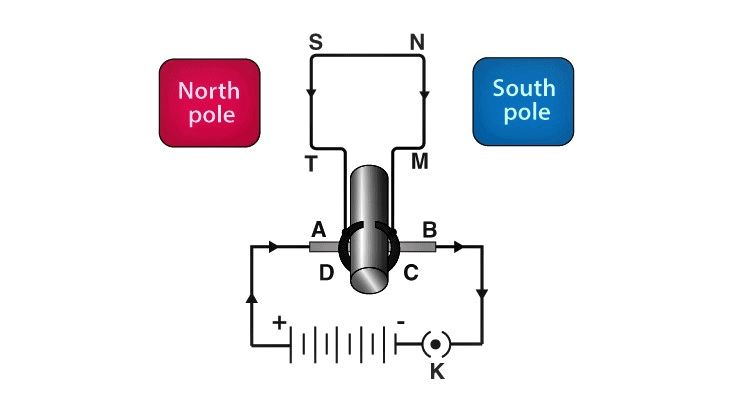Principle: It works on the principle of the magnetic effect of current. A current-carrying coil rotates in a magnetic field.
Working: When a current is allowed to flow through the coil MNST by closing the switch, the coil starts rotating anti-clockwise. This happens because a downward force acts on length MN and at the same time, an upward force acts on length ST. As a result, the coil rotates anti-clockwise.
Current in the length MN flows from M to N and the magnetic field acts from left to right, normal to length MN. Therefore, according to Fleming's left-hand rule, a downward force acts on the length of MN. Similarly, current in the length ST flows from S to T and the magnetic field acts from left to right, normal to the flow of current. Therefore, an upward force acts on the length ST. These two forces cause the coil to rotate anti-clockwise.
After half a rotation, the position of MN and ST interchange. The half-ring D comes in contact with brush A and half-ring C comes in contact with brush B. Hence, the direction of current in the coil MNST gets reversed.
The current flows through the coil in the direction TSNM. The reversal of current through the coil MNST repeats after each half rotation. As a result, the coil rotates unidirectionally.
The split rings help to reverse the direction of current in the circuit. These are called the commutator.

Q 12. Name some devices in which electric motors are used?
Some devices in which electric motors are Water pumps, Electric fans, Electric mixers and Washing machines.

Q 13. A coil of insulated copper wire is connected to a galvanometer. What will happen if a bar magnet is (i) pushed into the coil, (ii) withdrawn from inside the coil, (iii) held stationary inside the coil?

(i) When a bar magnet is pushed into the coil, current is induced in the coil momentarily as a result the galvanometer deflects in a particular direction momentarily.

(ii) When the bar magnet is withdrawn from inside the coil, current is induced momentarily but in the opposite direction and the galvanometer deflects in the opposite direction momentarily.

(iii) When the bar magnet is held stationary inside the coil, no current will be induced as a result there will be no deflection in the galvanometer.

Q 14. Two circular coils A and B are placed closed to each other. If the current in the coil A is changed, will some current be induced in coil B? Give reason.

Two circular coils A and B are placed close to each other. When the current in coil A is changed, the magnetic field associated with it also changes. As a result, the magnetic field around coil B also changes. This change in magnetic field lines around coil B induces an electric current in it. This is called electromagnetic induction.

Q 15. State the rule to determine the direction of a (i) magnetic field produced around a straight conductor carrying current, (ii) force experienced by a current-carrying straight conductor placed in a magnetic field which is perpendicular to it, and (iii) current induced in a coil due to its rotation in a magnetic field.

(i) The rule used to determine the direction of the magnetic field produced around a straight conductor-carrying current is Maxwell’s right-hand thumb rule.

(ii) The rule used to determine the force experienced by a current-carrying straight conductor placed in a magnetic field that is perpendicular to it is Fleming’s left-hand rule.

(iii) The rule used to determine the current induced in a coil due to its rotation in a magnetic field is Fleming’s right-hand rule.

Q 16. Explain the underlying principle and working of an electric generator by drawing a labelled diagram. What is the function of brushes?

Principle: An electric generator works on the principle of electromagnetic induction phenomenon. According to it, whenever a coil is rotated between the poles of a magnet, an induced current is set up in the coil, whose direction is given by Fleming’s right-hand rule.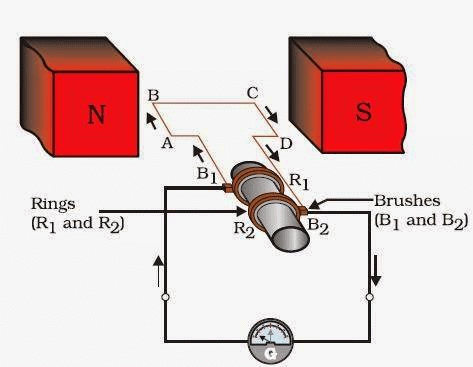Working: Let in the beginning, as shown in Fig. brushes B1 and B2 are kept pressed separately on rings R1 and R2 respectively. Let the axle attached to the rings is rotated such that arm AB of the coil moves up and arm CD moves down in the magnetic field. Due to rotation of arms AB and CD induced currents are set up in them. As per Fleming’s right-hand rule induced currents in these arms are along with the directions AB and CD. Thus an induced current flows along ABCD and current in the external circuit flows from B2 to B1.
After half a rotation, arm AB starts moving down and the arm CD upward. Therefore, directions of induced currents in these arms change. Thus net induced current now becomes in the direction DCBA. In the external circuit now current flows from B1 to B2. Thus after every half rotation current changes its direction and an alternating current is obtained from the generator.
The function of Brushes: Brushes are kept pressed on the two slip rings separately. The outer ends of the brushes are connected to the galvanometer (or the external load). Thus brushes help in transferring current from the coil ABCD to the external circuit.

Q 17. When does an electric short circuit occur?
Listed below are two instances of when a short-circuit can occur:

(i) When too many appliances are connected to a single socket or when high power rating appliances are connected to a light circuit, the resistance of the circuit becomes low as a result the current flowing through the circuit becomes very high. This condition results in a short circuit.

(ii) When live wires whose insulation have worn off come in contact with each other, the current flowing in the circuit increases abruptly which results in a short circuit.

Q 18. What is the function of an earth wire? Why is it necessary to earth metallic appliances?
The metallic body of electric appliances is connected to the earth by means of earth wire so that any leakage of electric current is transferred to the ground. This prevents any electric shock to the user. That is why earthing of the electrical appliances is necessary.

The document NCERT Solutions: Magnetic Effect on Electric Current Notes | Study Science Class 10 - Class 10 is a part of the Class 10 Course Science Class 10.
All you need of Class 10 at this link: Class 10Use Code STAYHOME200 and get INR 200 additional OFF

## Science Class 10

107 videos|325 docs|162 tests

Track your progress, build streaks, highlight & save important lessons and more!

,

,

,

,

,

,

,

,

,

,

,

,

,

,

,

,

,

,

,

,

,

;